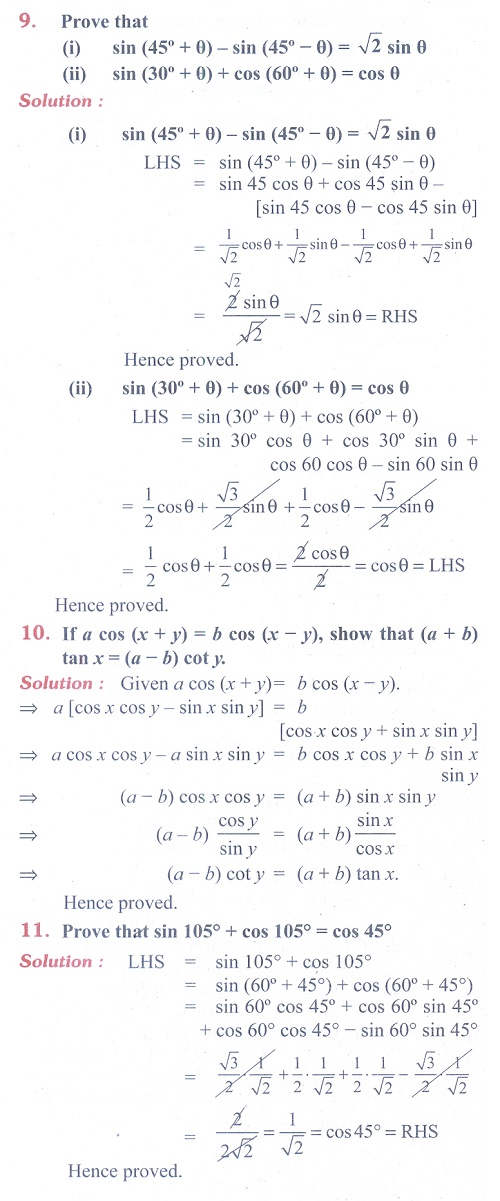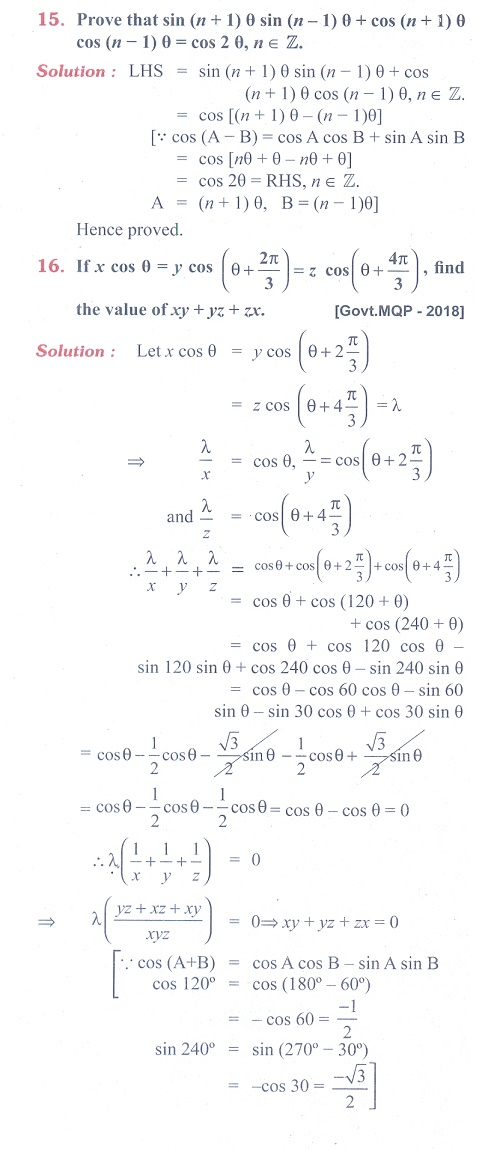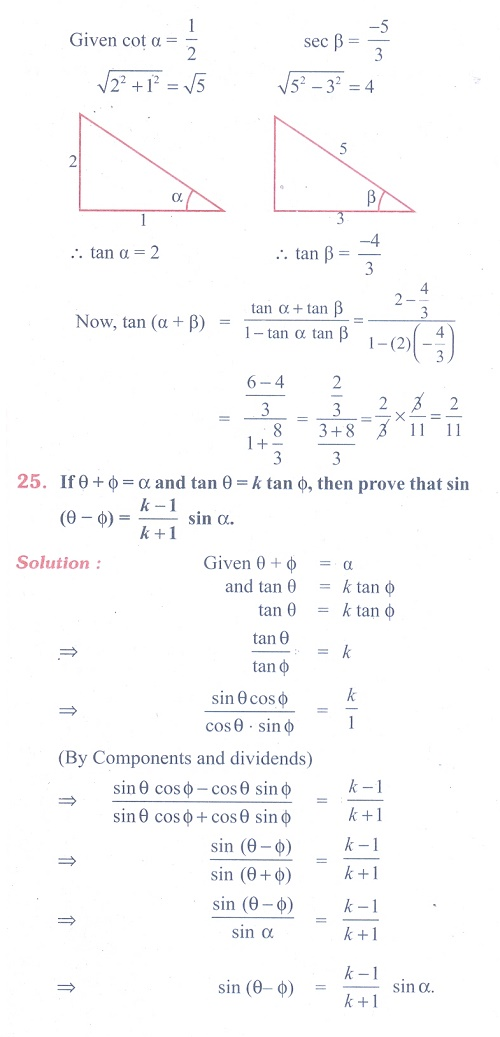Home | | Maths 11th std | Exercise 3.4: Trigonometric Identities: Sum and difference identities or compound angles formulas

# Exercise 3.4: Trigonometric Identities: Sum and difference identities or compound angles formulas

Maths Book back answers and solution for Exercise questions - Mathematics : Trigonometry: Trigonometric Identities: Sum and difference identities or compound angles formulasTags : Problem Questions with Answer, Solution | Mathematics , 11th Mathematics : UNIT 3 : Trigonometry
Study Material, Lecturing Notes, Assignment, Reference, Wiki description explanation, brief detail
11th Mathematics : UNIT 3 : Trigonometry : Exercise 3.4: Trigonometric Identities: Sum and difference identities or compound angles formulas | Problem Questions with Answer, Solution | Mathematics